Publicité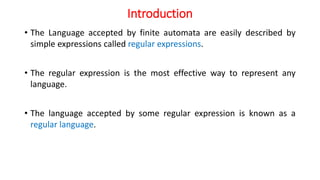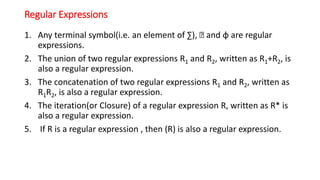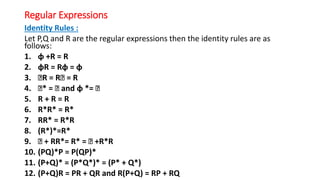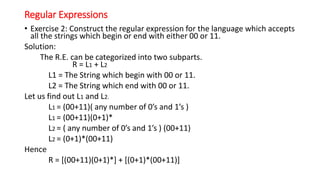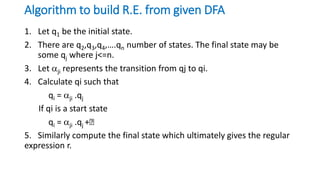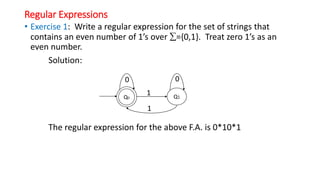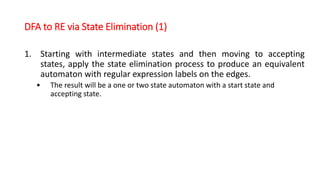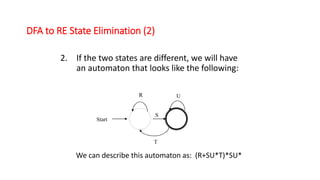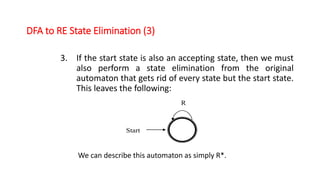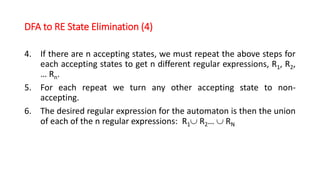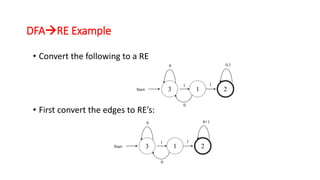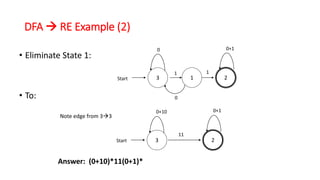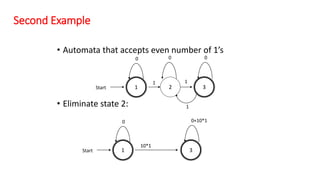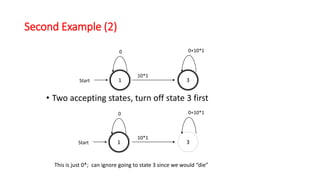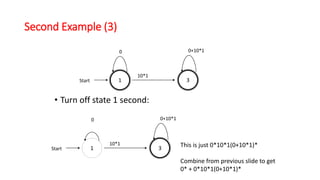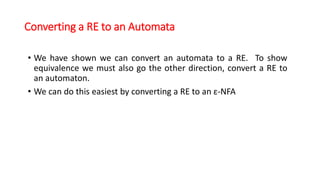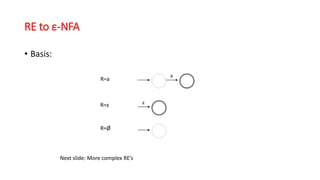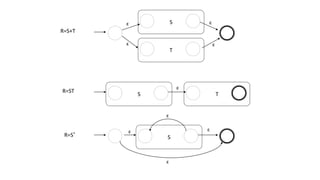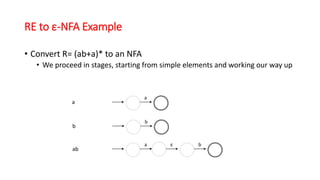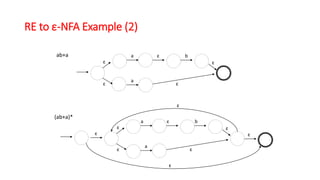1 sur 22
Publicité

### Regular expressions

1. REGULAR EXPRESSIONS M RATNAKAR BABU Asst.Prof. CSE
2. Introduction • The Language accepted by finite automata are easily described by simple expressions called regular expressions. • The regular expression is the most effective way to represent any language. • The language accepted by some regular expression is known as a regular language.
3. Regular Expressions 1. Any terminal symbol(i.e. an element of ∑), Ꜫ and φ are regular expressions. 2. The union of two regular expressions R1 and R2, written as R1+R2, is also a regular expression. 3. The concatenation of two regular expressions R1 and R2, written as R1R2, is also a regular expression. 4. The iteration(or Closure) of a regular expression R, written as R* is also a regular expression. 5. If R is a regular expression , then (R) is also a regular expression.
4. Identity Rules : Let P,Q and R are the regular expressions then the identity rules are as follows: 1. φ +R = R 2. φR = Rφ = φ 3. ꜪR = RꜪ = R 4. Ꜫ* = Ꜫ and φ *= Ꜫ 5. R + R = R 6. R*R* = R* 7. RR* = R*R 8. (R*)*=R* 9. Ꜫ + RR*= R* = Ꜫ +R*R 10. (PQ)*P = P(QP)* 11. (P+Q)* = (P*Q*)* = (P* + Q*) 12. (P+Q)R = PR + QR and R(P+Q) = RP + RQ Regular Expressions
5. • Exercise 2: Construct the regular expression for the language which accepts all the strings which begin or end with either 00 or 11. Solution: The R.E. can be categorized into two subparts. R = L1 + L2 L1 = The String which begin with 00 or 11. L2 = The String which end with 00 or 11. Let us find out L1 and L2. L1 = (00+11)( any number of 0’s and 1’s ) L1 = (00+11)(0+1)* L2 = ( any number of 0’s and 1’s ) (00+11) L2 = (0+1)*(00+11) Hence R = [(00+11)(0+1)*] + [(0+1)*(00+11)] Regular Expressions
6. Algorithm to build R.E. from given DFA 1. Let q1 be the initial state. 2. There are q2,q3,q4,….qn number of states. The final state may be some qj where j<=n. 3. Let ji represents the transition from qj to qi. 4. Calculate qi such that qi = ji .qj If qi is a start state qi = ji .qj +Ꜫ 5. Similarly compute the final state which ultimately gives the regular expression r.
7. • Exercise 1: Write a regular expression for the set of strings that contains an even number of 1’s over ={0,1}. Treat zero 1’s as an even number. Solution: The regular expression for the above F.A. is 0*10*1 Regular Expressions Q0 Q1 1 0 0 1
8. DFA to RE via State Elimination (1) 1. Starting with intermediate states and then moving to accepting states, apply the state elimination process to produce an equivalent automaton with regular expression labels on the edges. • The result will be a one or two state automaton with a start state and accepting state.
9. DFA to RE State Elimination (2) 2. If the two states are different, we will have an automaton that looks like the following: Start S R T U We can describe this automaton as: (R+SU*T)*SU*
10. DFA to RE State Elimination (3) 3. If the start state is also an accepting state, then we must also perform a state elimination from the original automaton that gets rid of every state but the start state. This leaves the following: Start R We can describe this automaton as simply R*.
11. DFA to RE State Elimination (4) 4. If there are n accepting states, we must repeat the above steps for each accepting states to get n different regular expressions, R1, R2, … Rn. 5. For each repeat we turn any other accepting state to non- accepting. 6. The desired regular expression for the automaton is then the union of each of the n regular expressions: R1 R2…  RN
12. DFARE Example • Convert the following to a RE • First convert the edges to RE’s: 3Start 1 2 1 1 0 0 0,1 3Start 1 2 1 1 0 0 0+1
13. DFA  RE Example (2) • Eliminate State 1: • To: Start 0 0 3 1 2 1 1 0+1 3Start 2 11 0+10 0+1 Note edge from 33 Answer: (0+10)*11(0+1)*
14. Second Example • Automata that accepts even number of 1’s • Eliminate state 2: 1Start 2 3 1 1 0 1 00 0+10*1 1Start 3 0 10*1
15. Second Example (2) • Two accepting states, turn off state 3 first 1Start 3 0 0+10*1 10*1 This is just 0*; can ignore going to state 3 since we would “die” 1Start 0 3 0+10*1 10*1
16. Second Example (3) • Turn off state 1 second: 1Start 3 0 0+10*1 10*1 This is just 0*10*1(0+10*1)* Combine from previous slide to get 0* + 0*10*1(0+10*1)* 0+10*1 1Start 3 0 10*1
17. Converting a RE to an Automata • We have shown we can convert an automata to a RE. To show equivalence we must also go the other direction, convert a RE to an automaton. • We can do this easiest by converting a RE to an ε-NFA
18. RE to ε-NFA • Basis: R=a R=ε ε R=Ø a Next slide: More complex RE’s
19. R=S+T S T ε ε ε ε R=ST S T ε R=S* ε S ε ε ε
20. RE to ε-NFA Example • Convert R= (ab+a)* to an NFA • We proceed in stages, starting from simple elements and working our way up a a b b ab a bε
21. RE to ε-NFA Example (2) ab+a (ab+a)* a bε a ε ε ε ε a bε a ε ε ε ε εε ε ε
22. End for Now Continue in Next Class
Publicité